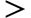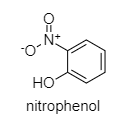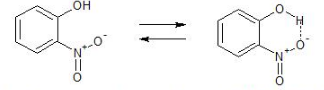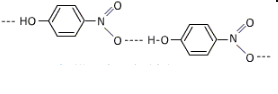QuestionAnswers

# The correct order of decreasing acidity of nitrophenols will be:a. m-nitrophenolp-nitrophenolo-nitrophenolb. o-nitrophenolm-nitrophenolp-nitrophenolc. p-nitrophenolm-nitrophenolo-nitrophenold. p-nitrophenolo-nitrophenolm-nitrophenolVerified
56.8k+ views
Hint: The general formula of nitrophenols is: ${ HOC }_{ 6 }{ H }_{ 5-x }{ (NO }_{ 2 }{ ) }_{ x }$
The structure of nitrophenol is shown below:Here the acidity depends on the presence of electron-withdrawing or electron releasing groups and their respective positions.

An inductive effect is an electronic effect due to the polarisation of σ bonds within a molecule or ion. This is typically due to an electronegativity difference between the atoms at either end of the bond.
${ -NO }_{ 2 }$ is an electron-withdrawing group.
${ -NO }_{ 2 }$ group at ortho and para position pulls back electrons of the OH bond towards itself by the stronger -R effect while the ${ -NO }_{ 2 }$ group at m-position pulls back electrons of the OH bond by the weaker effect.
Thus, ortho and para-nitrophenols are more acidic than m-nitrophenol is a little less acidic than p-nitrophenol because of intramolecular hydrogen bonding which makes the loss of a proton difficult to remove.Figure: Showing intramolecular hydrogen bonding in m-nitrophenol.Figure: Showing intermolecular hydrogen bonding in p-nitrophenol

Hence, The correct order of decreasing acidity of nitrophenols will be:
p-nitrophenolo-nitrophenolm-nitrophenol
The correct option is D.